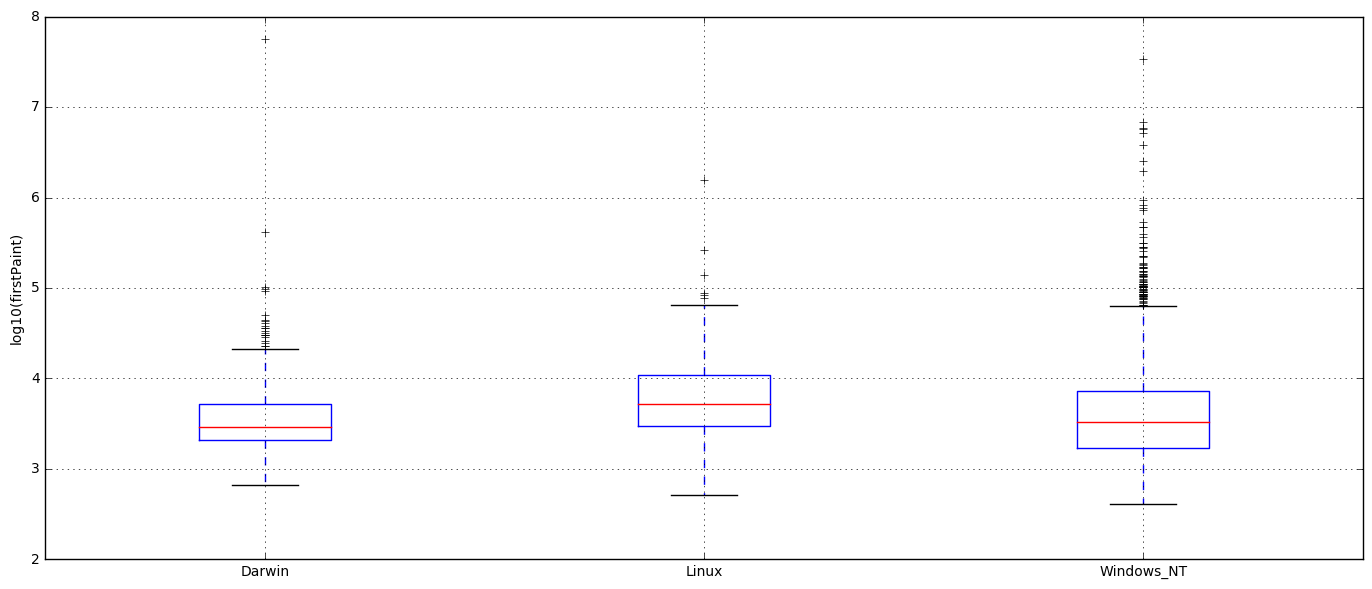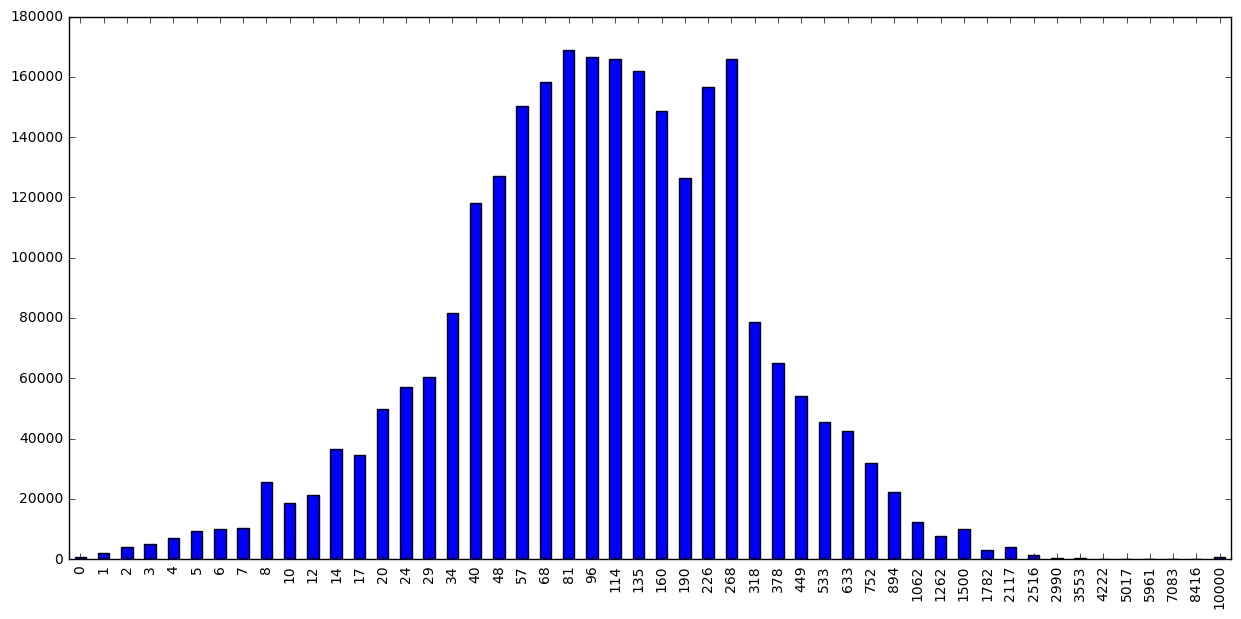### Telemetry Hello World

This is a very a brief introduction to Spark and Telemetry in Python. You should have a look at the tutorial in Scala and the associated talk if you are interested to learn more about Spark.

import ujson as json
import matplotlib.pyplot as plt
import pandas as pd
import numpy as np
import plotly.plotly as py

from plotly.graph_objs import *
from moztelemetry import get_pings_properties, get_one_ping_per_client
from moztelemetry.dataset import Dataset

%matplotlib inline

Unable to parse whitelist (/home/hadoop/anaconda2/lib/python2.7/site-packages/moztelemetry/histogram-whitelists.json). Assuming all histograms are acceptable.


### Basics

The goal of this example is to plot the startup distribution for each OS. Let’s see how many parallel workers we have at our disposal:

sc.defaultParallelism

32


Let’s fetch 10% of Telemetry submissions for a given submission date…

Dataset.from_source("telemetry").schema

[u'submissionDate',
u'sourceName',
u'sourceVersion',
u'docType',
u'appName',
u'appUpdateChannel',
u'appVersion',
u'appBuildId']

pings = Dataset.from_source("telemetry") \
.where(docType='main') \
.where(submissionDate="20161101") \
.where(appUpdateChannel="nightly") \
.records(sc, sample=0.1)


… and extract only the attributes we need from the Telemetry submissions:

subset = get_pings_properties(pings, ["clientId",
"environment/system/os/name",


Let’s filter out submissions with an invalid startup time:

subset = subset.filter(lambda p: p.get("payload/simpleMeasurements/firstPaint", -1) >= 0)


To prevent pseudoreplication, let’s consider only a single submission for each client. As this step requires a distributed shuffle, it should always be run only after extracting the attributes of interest with get_pings_properties.

subset = get_one_ping_per_client(subset)


Caching is fundamental as it allows for an iterative, real-time development workflow:

cached = subset.cache()


How many pings are we looking at?

cached.count()

7132


Let’s group the startup timings by OS:

grouped = cached.map(lambda p: (p["environment/system/os/name"], p["payload/simpleMeasurements/firstPaint"])).groupByKey().collectAsMap()


And finally plot the data:

frame = pd.DataFrame({x: np.log10(pd.Series(list(y))) for x, y in grouped.items()})
plt.figure(figsize=(17, 7))
frame.boxplot(return_type="axes")
plt.ylabel("log10(firstPaint)")
plt.show()You can also create interactive plots with plotly:

fig = plt.figure(figsize=(18, 7))
frame["Windows_NT"].plot(kind="hist", bins=50)
plt.title("startup distribution for Windows")
plt.ylabel("count")
plt.xlabel("log10(firstPaint)")
py.iplot_mpl(fig, strip_style=True)


### Histograms

Let’s extract a histogram from the submissions:

histograms = get_pings_properties(pings, "payload/histograms/GC_MARK_MS", with_processes=True)


The API returns three distinct histograms for each submission: - a histogram for the parent process (GC_MARK_MS_parent) - an aggregated histogram for the child processes (GC_MARK_MS_children) - the aggregate of the parent and child histograms (GC_MARK)

Let’s aggregate the histogram over all submissions and plot it:

def aggregate_arrays(xs, ys):
if xs is None:
return ys

if ys is None:
return xs

return xs + ys

aggregate.plot(kind="bar", figsize=(15, 7))

<matplotlib.axes._subplots.AxesSubplot at 0x7f1cea8b49d0>Keyed histograms follow a similar pattern. To extract a keyed histogram for which we know the key/label we are interested in:

histograms = get_pings_properties(pings, "payload/keyedHistograms/SUBPROCESS_ABNORMAL_ABORT/plugin", with_processes=True)


List all keys/labels for a keyed histogram:

keys = pings.flatMap(lambda p: p["payload"].get("keyedHistograms", {}).get("MISBEHAVING_ADDONS_JANK_LEVEL", {}).keys())
keys = keys.distinct().collect()

keys[:5]

[u'firefox@zenmate.com',
u'jid1-f3mYMbCpz2AZYl@jetpack',
u'jid0-SQnwtgW1b8BsMB5PLV5WScEDWOjw@jetpack',
u'light_plugin_ACF0E80077C511E59DED005056C00008@kaspersky.com',
u'netvideohunter@netvideohunter.com']


Retrieve the histograms for a set of labels:

properties = map(lambda k: "payload/keyedHistograms/{}/{}".format("MISBEHAVING_ADDONS_JANK_LEVEL", k), keys[:5])

histograms = get_pings_properties(pings, properties, with_processes=True)